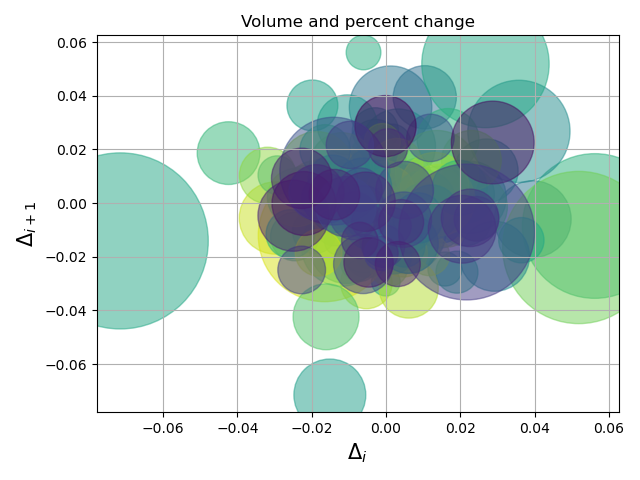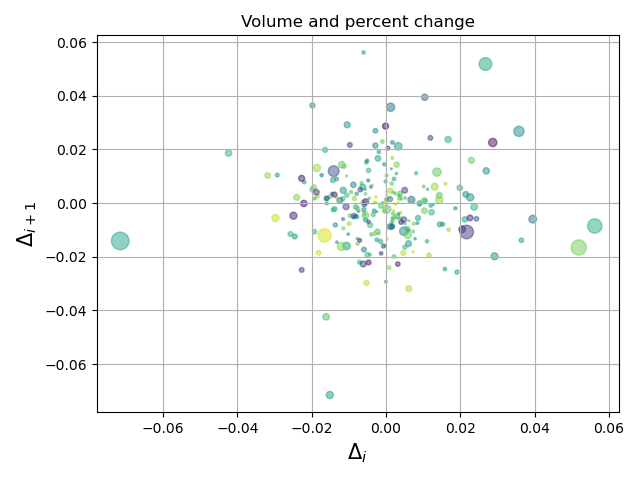# Get_sample_data() got an unexpected keyword argument 'np_load'

Hey guys, I am following the scatter plot code here. Then it ran into error `get_sample_data() got an unexpected keyword argument 'np_load'`.

I am using numpy version 1.15.0 & matplotlib 3.0.3. Thank you in advance.

Welcome HaFred !!!

Could you post the code ?

Rgds.

``````import numpy as np
import matplotlib.pyplot as plt
import matplotlib.cbook as cbook

# Load a numpy record array from yahoo csv data with fields date, open, close,
# volume, adj_close from the mpl-data/example directory. The record array
# stores the date as an np.datetime64 with a day unit ('D') in the date column.
.view(np.recarray))
price_data = price_data[-250:]  # get the most recent 250 trading days

# Marker size in units of points^2
volume = (15 * price_data.volume[:-2] / price_data.volume)**2
close = 0.003 * price_data.close[:-2] / 0.003 * price_data.open[:-2]

fig, ax = plt.subplots()
ax.scatter(delta1[:-1], delta1[1:], c=close, s=volume, alpha=0.5)

ax.set_xlabel(r'\$\Delta_i\$', fontsize=15)
ax.set_ylabel(r'\$\Delta_{i+1}\$', fontsize=15)
ax.set_title('Volume and percent change')

ax.grid(True)
fig.tight_layout()

plt.show()
``````

FYI, basically I just follow the matplotlib document. Thanks a lot.

Well I could not figure out this error, my installation of Matplotlib (Anaconda) is not working very well - I cannot even get the last version of Matplotlib.

But I got the data in another way using Pandas Datareader :

import numpy as np
import matplotlib.pyplot as plt

price_data = price_data[-250:] # get the most recent 250 trading days
delta1 = np.diff(price_data.Close) / price_data.Close[:-1]

volume = (15 * price_data.Volume[:-2] / price_data.Volume)**2
close = 0.003 * price_data.Close[:-2] / 0.003 * price_data.Open[:-2]

fig, ax = plt.subplots()
ax.scatter(delta1[:-1], delta1[1:], c=close, s=volume, alpha=0.5)
ax.set_xlabel(r’\Delta_i’, fontsize=15)
ax.set_ylabel(r’\Delta_{i+1}’, fontsize=15)
ax.set_title(‘Volume and percent change’)
ax.grid(True)
fig.tight_layout()
plt.show()

But there are some differences : This database does no have adjusted close price, so I used the close price.

This is not what you request, but I hope it might help.

For sure later this error message will be properly solved.

Rgds.Thanks a lot, El_Uatu! This seems like a zoom in plot for GOOG data, I am wondering do you know how to zoom out and make the range for both x, y axis larger like in the document?

1 Like

Well, since I cannot see the data of the original plot to compare to the data used, I guessed and divided the volume (size) per 100.

ax.scatter(delta1[:-1], delta1[1:], c=close, s=volume/100, alpha=0.5)

And got :I think you need to either update to matplotlib v3.3 or remove the `np_load` kwargs from your local copy of the example

I could not find anything like " np_load= True " that can be given as an attribute, so your code should something like: